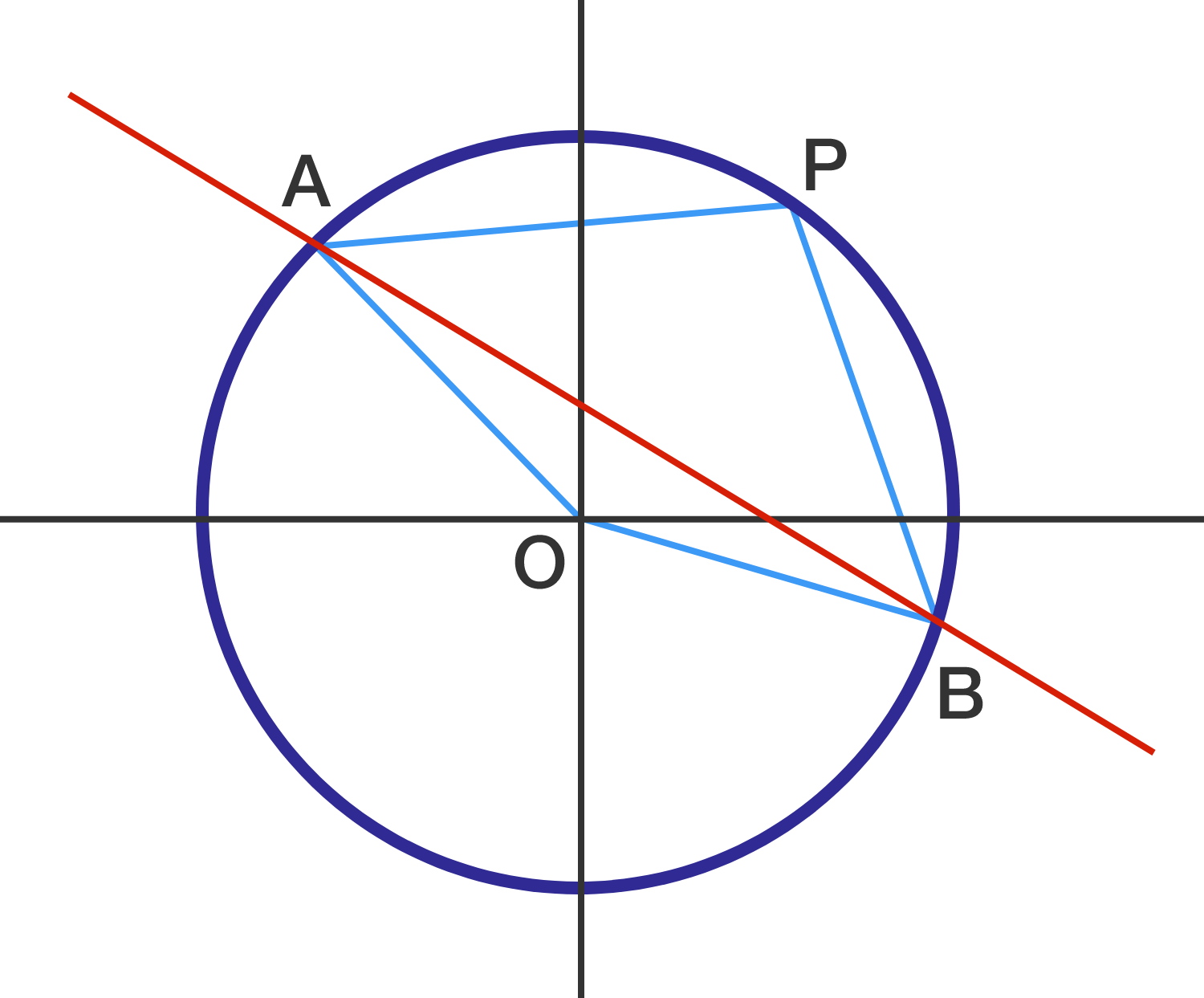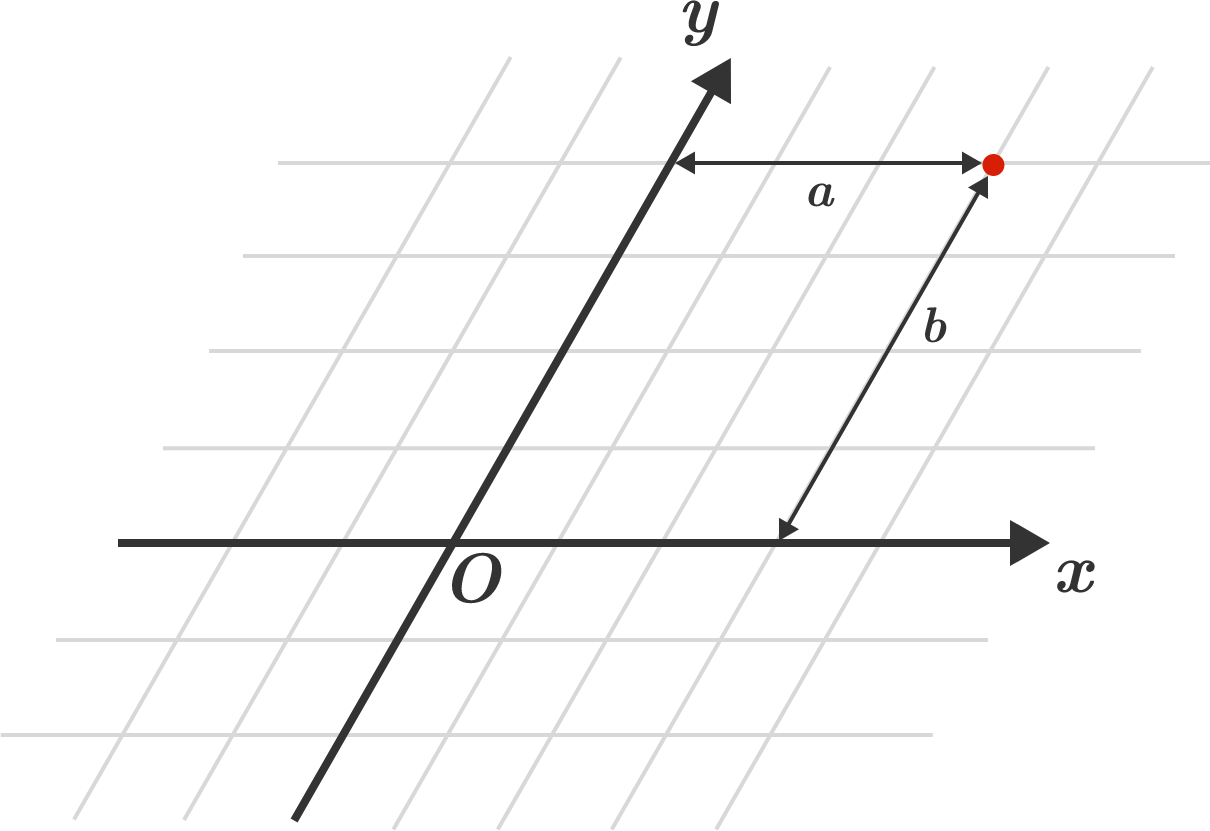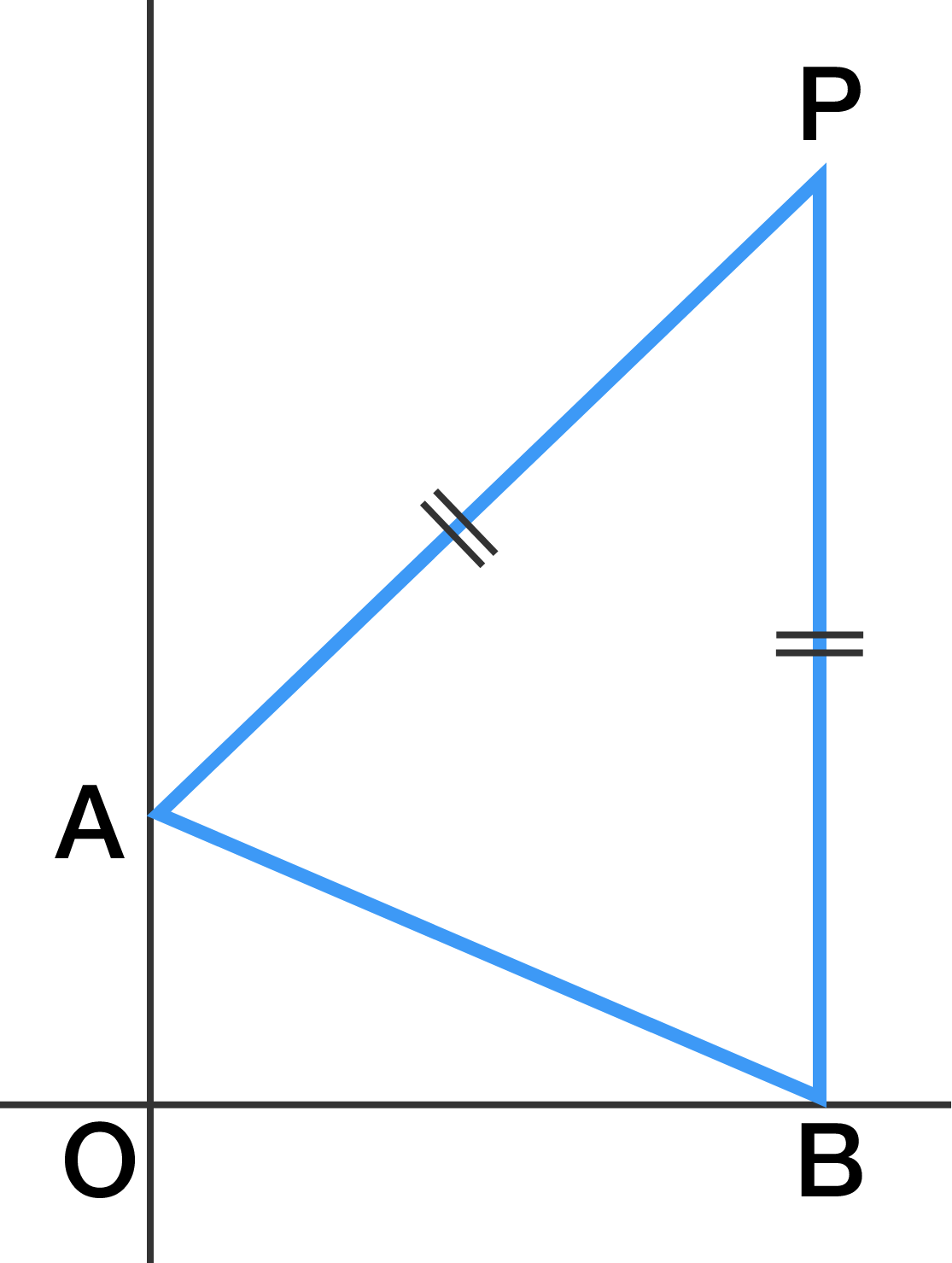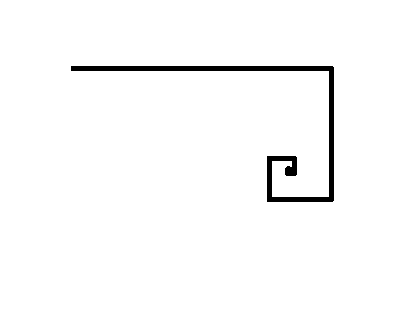Geometry

# 2D Coordinate Geometry: Level 3 ChallengesCircle $O$ is centered at the point of origin with point $P = (3 , 4)$ lying on it. The red line $l : 3x + 4y - 7 = 0$ intersects the circle at points $A$ and $B,$ as shown.

What is the area of quadrilateral $AOBP?$

$\large {\left\{\begin{matrix}A_{n+1}=\alpha A_n+(1-\alpha)B_n \\ B_{n+1}=\alpha B_n+(1-\alpha)C_n \\ C_{n+1}=\alpha C_n+(1-\alpha)A_n\end{matrix}\right.}$

Let $A_1,B_1,C_1$ be three distinct non-collinear points on the coordinate plane.
Also $A_n,B_n,C_n$ satisfy the recurrence relation above ($0<\alpha<1$).

Then $\displaystyle\lim_{n\to\infty}A_n$ is the $\text{\_\_\_\_\_\_\_\_\_\_}$ of the triangle $A_1B_1C_1$.Did you know that we can have coordinate systems where the coordinate axes are not perpendicular to each other? Such coordinate systems are known as oblique coordinate systems.

The above figure shows an oblique coordinate system. In such systems, the $x$-coordinate of a point is found by measuring the distance from the point to the $y$-axis parallel to the $x$-axis. Similarly, the $y$-coordinate of a point is found by measuring the distance from the point to the $x$-axis parallel to the $y$-axis. The red point in the above figure has the coordinates $(a, b)$.

Question: We are given two points: $A \ (1,6)$ and $B \ (5,2)$ in an oblique coordinate system where the angle between the positive axes is $60^{\circ}$. What is the distance between the two points, $AB$?The $\triangle AOB$ consists of point $A = (0 , 1)$, point $O = (0 , 0)$, and point $B$ lying somewhere on the $x$-axis.

Let $P$ be the point in the first quadrant such that $AP = PB$ and $AO \parallel PB$, as shown above.

If the length of $OP$ is $\sqrt{194}$, what is the area of the quadrilateral $AOBP?$A particle is on the point $(3,5)$ on the plane. It goes 10 units in the direction of positive $x$-axis. Then it turns towards right making an angle of 90 degrees with its initial direction and goes ahead 5 units, Now it again takes a right turn and goes ahead 2.5 units. It keeps turning right and travels half the distance traveled in the turn just before it . This goes on without end. Find the final distance of the particle from the origin $(0,0)$?

The answer would come in the form square root of $x$, submit your answer as $x$.

×